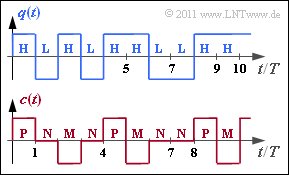# Exercise 1.4: Entropy Approximations for the AMI CodeBinary source signal (top) and
ternary encoder signal (bottom)

The graph above shows the binary source signal  $q(t)$, which can also be described by the symbol sequence  $\langle q_\nu \rangle$  with  $q_\nu \in \{ {\rm L}, {\rm H} \}$ .  In the entire task,  $p_{\rm L} = p_{\rm H} =0.5$ applies.

The coded signal  $c(t)$  and the corresponding symbol sequence  $\langle c_\nu \rangle$  with  $c_\nu \in \{{\rm P}, {\rm N}, {\rm M} \}$  results from the AMI coding ("Alternate Mark Inversion") according to the following rule:

• The binary symbol  $\rm L$  ⇒  "Low"is always represented by the ternary symbol  $\rm N$  ⇒  "German: Null" ⇒ "Zero".
• The binary symbol  $\rm H$  ⇒  "High"  is also encoded deterministically but alternately (hence the name "Alternate Mark Inversion") by the symbols  $\rm P$  ⇒  "Plus"  and  $\rm M$  ⇒  "Minus".

In this task, the entropy approximations for the AMI coded signal are to be calculated:

• Approximation  $H_1$  refers only to the symbol probabilities  $p_{\rm P}$,  $p_{\rm N}$  and  $p_{\rm M}$.
• The  $k$–th is the entropy approximation  $(k = 2, 3, \text{...} \ )$  can be determined according to the following equation:
$$H_k = \frac{1}{k} \cdot \sum_{i=1}^{3^k} p_i^{(k)} \cdot {\rm log}_2\hspace{0.1cm}\frac {1}{p_i^{(k)}} \hspace{0.5cm}({\rm Einheit\hspace{-0.1cm}: \hspace{0.1cm}bit/Symbol}) \hspace{0.05cm}.$$
Here,  $p_i^{(k)}$  the  $i$–th composite probability of a  $k$–tuple.

Hints:

• This task belongs to the chapter  Discrete Sources with Memory.
• Reference is made in particular to the page  The Entropy of the AMI code.
• In  Exercise 1.4Z  the actual entropy of the code symbol sequence  $\langle c_\nu \rangle$  is calculated to  $H = 1 \; \rm bit/symbol$.
• The following relations between the units are to be expected:   $H \le$ ...$\le H_3 \le H_2 \le H_1 \le H_0 \hspace{0.05cm}.$

### Questions

1

What is the decision content of the AMI code?

 $H_0 \ = \$ $\ \rm bit/symbol$

2

Calculate the first entropy approximation of the AMI code.

 $H_1 \ = \$ $\ \rm bit/symbol$

3

What is the entropy approximation  $H_2$, based on two-tuples?

 $H_2 \ = \$ $\ \rm bit/symbol$

4

What is the value of the entropy approximation  $H_3$, based on three-tuples?

 $H_3 \ = \$ $\ \rm bit/symbol$

5

Which statements apply to the entropy approximation  $H_4$?

 It must be averaged over  $3^4 = 81$  summands. $1 \; {\rm bit/symbol} < H_4 < H_3$ is valid. After a long calculation you get  $H_4 = 1.333 \; {\rm bit/symbol}$.

### Solution

#### Solution

(1)  The symbol set size is  $M = 3$.  This gives the decision content with the  logarithm  to the base $2$   ⇒   $\log_2$:

$$H_0 = {\rm log}_2\hspace{0.1cm} M = {\rm log}_2\hspace{0.1cm} (3) \hspace{0.15cm} \underline { = 1.585 \,{\rm bit/symbol}} \hspace{0.05cm}.$$

(2)  The first-order entropy approximation takes into account only the symbol probabilities  $p_{\rm P}$,  $p_{\rm N}$  and  $p_{\rm M}$
and not the statistical bindings within the code sequence  $\langle c_\nu \rangle$.  Thus one obtains:

$$p_{\rm N} = p_{\rm L} = 1/2\hspace{0.05cm},\hspace{0.2cm}p_{\rm P} = p_{\rm M} = p_{\rm H}/2 = 1/4 \hspace{0.3cm} \Rightarrow\hspace{0.3cm} H_1 = \frac{1}{2} \cdot {\rm log}_2\hspace{0.1cm} (2) + 2 \cdot \frac{1}{4} \cdot {\rm log}_2\hspace{0.1cm}(4) \hspace{0.15cm} \underline {= 1.5 \,{\rm bit/Symbol}} \hspace{0.05cm}.$$

(3)  First, the  $M^2 = 9$  composite probabilities of two-tuples have to be determined here, in the following marked by the first two code symbols  $c_1$  and  $c_2$:

• Since in the AMI code neither  $\rm P$  can follow  $\rm P$  nor  $\rm M$  can follow  $\rm M$ ,  $p_{\rm PP} = p_{\rm MM} =0$.
• For the composite probabilities under the condition  $c_2 = \rm N$  applies:
$$p_{\rm NN} \hspace{0.1cm} = \hspace{0.1cm} {\rm Pr}( c_1 = \mathbf{N}) \cdot {\rm Pr}(c_2 = \mathbf{N}\hspace{0.05cm} | c_1 = \mathbf{N}) = 1/2 \cdot 1/2 = 1/4 \hspace{0.05cm},$$
$$p_{\rm MN} \hspace{0.1cm} = \hspace{0.1cm} {\rm Pr}( c_1 = \mathbf{M}) \cdot {\rm Pr}(c_2 = \mathbf{N}\hspace{0.05cm} | c_1 = \mathbf{M}) = 1/4 \cdot 1/2 = 1/8 \hspace{0.05cm},$$
$$p_{\rm PN} \hspace{0.1cm} = \hspace{0.1cm} {\rm Pr}( c_1 = \mathbf{P}) \cdot {\rm Pr}(c_2 = \mathbf{N}\hspace{0.05cm} | c_1 = \mathbf{P}) = 1/4 \cdot 1/2 = 1/8 \hspace{0.05cm}.$$
• The composite probabilities of the two-tuples  $\rm PM$  and  $\rm MP$  are:
$$p_{\rm PM} \hspace{0.1cm} = \hspace{0.1cm} {\rm Pr}( c_1 = \mathbf{P}) \cdot {\rm Pr}(c_2 = \mathbf{M}\hspace{0.05cm} | c_1 = \mathbf{P}) = 1/4 \cdot 1/2 = 1/8 \hspace{0.05cm},$$
$$p_{\rm MP} \hspace{0.1cm} = \hspace{0.1cm} {\rm Pr}( c_1 = \mathbf{M}) \cdot {\rm Pr}(c_2 = \mathbf{P}\hspace{0.05cm} | c_1 = \mathbf{M}) = 1/4 \cdot 1/2 = 1/8 \hspace{0.05cm}.$$
• For the remaining probabilities, it must also be taken into account whether the binary symbol  $\rm H$  was encoded with  $\rm P$  or with  $\rm M$  last time  ⇒  further factor  $1/2$:
$$p_{\rm NM} \hspace{0.1cm} = \hspace{0.1cm} {\rm Pr}( c_1 = \mathbf{N}) \cdot {\rm Pr}(c_2 = \mathbf{M}\hspace{0.05cm} | c_1 = \mathbf{N}) = 1/2 \cdot 1/2 \cdot 1/2= 1/8 \hspace{0.05cm},$$
$$p_{\rm NP} \hspace{0.1cm} = \hspace{0.1cm} {\rm Pr}( c_1 = \mathbf{N}) \cdot {\rm Pr}(c_2 = \mathbf{P}\hspace{0.05cm} | c_1 = \mathbf{N}) = 1/2 \cdot 1/2 \cdot 1/2 = 1/8 \hspace{0.05cm}.$$

Thus the entropy  $H_2'$  of a two-tuple or its entropy  $H_2$  per code symbol:

$$H_2\hspace{0.01cm}' = \frac{1}{4} \cdot {\rm log}_2\hspace{0.1cm} (4) + 6 \cdot \frac{1}{8} \cdot {\rm log}_2\hspace{0.1cm}(8) \hspace{0.15cm} {= 2.75 \,{\text{bit/two-tuple} }}\hspace{0.3cm} \Rightarrow\hspace{0.3cm} H_2 = \frac{H_2\hspace{0.01cm}'}{2} \hspace{0.15cm} \underline {= 1.375 \,{\rm bit/symbol}} \hspace{0.05cm}.$$

(4)  The calculation of  $H_3$  is similar to the last subtask for  $H_2$, except that now  $3^3 = 27$  composite probabilities must be determined:

$$p_{\rm NNN} = 1/8\hspace{0.4cm}{\rm (nur \hspace{0.15cm}only once)} \hspace{0.05cm},$$
$$p_{\rm NMM} = p_{\rm NPP} = p_{\rm MNM} = ... = 0 \hspace{0.4cm}{\rm (total \hspace{0.15cm}12)} \hspace{0.05cm},$$
$$p_{\rm NNM} = p_{\rm NNP} = p_{\rm PMP} = ... = 1/16 \hspace{0.4cm}{\rm (total \hspace{0.15cm}14)}$$
$$\Rightarrow\hspace{0.3cm} H_3 = \frac{1}{3} \cdot \left [ \frac{1}{8} \cdot {\rm log}_2\hspace{0.1cm} (8) + 14 \cdot \frac{1}{16} \cdot {\rm log}_2\hspace{0.1cm}(16) \right ] \hspace{0.15cm} \underline {= 1.292 \,{\rm bit/symbol}} \hspace{0.05cm}.$$

(5)  Correct are the proposed solutions 1 and 2.

• On the other hand, statement 3 is wrong, because  $H_4$  must in any case be smaller than  $H_3 = 1.292 \; \rm bit/symbol$.